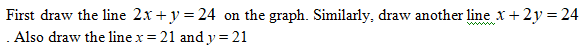# Hi, how would I go about solving this problem and what would the solution be?

Question
16 views

Hi, how would I go about solving this problem and what would the solution be?

check_circle

Step 1Step 2

Plot the points (0, 24), (12, 0), (0,12) and (24, 0). The commonly shaded region can be obtained by the inequality sign given.

The commonly shaded region will be surrounded by the points (1.5, 21), (21,21), (21, 1.5) and (8,8).

Step 3

After finding the feasible region, substitute the above obta...

### Want to see the full answer?

See Solution

#### Want to see this answer and more?

Solutions are written by subject experts who are available 24/7. Questions are typically answered within 1 hour.*

See Solution
*Response times may vary by subject and question.
Tagged in

### Other